Updating search results...

# 26 Results

View
Selected filters:
• anglesConditional Remix & Share Permitted
CC BY-NC-SA
Rating
0.0 stars

This resource includes tasks aligned to 4.MD.6.

Subject:
Mathematics
Material Type:
Activity/Lab
Formative Assessment
Author:
Drew Polly
07/25/2020Conditional Remix & Share Permitted
CC BY-NC-SA
Rating
0.0 stars

After reviewing types of angles and how to measure angles using a protractor, students will see how many triangles they can construct using an 18-foot beam.&nbsp; Through this activity, students will discover the conditions that must be met to construct a triangle.&nbsp; For example, the sum of the lengths of two sides of a triangle must be greater than the longest side of the triangle.&nbsp; They will also discover that the sum of the angles of a triangle is always 180 degrees.

Subject:
Mathematics
Material Type:
Activity/Lab
Author:
William Allred
Carrie Robledo
05/06/2021Rating
0.0 stars

Students must set the correct angle on the rescue launcher in order to rescue the aliens in this interactive game. Students are on a mission to rescue aliens and help them find their new space colony, Planet Geometry.

Subject:
Mathematics
Material Type:
Interactive
Provider:
Math Playground
Author:
Math Playground
02/26/2019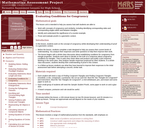Only Sharing Permitted
CC BY-NC-ND
Rating
0.0 stars

This lesson unit is intended to help teachers assess how well students are able to: work with concepts of congruency and similarity, including identifying corresponding sides and corresponding angles within and between triangles; Identify and understand the significance of a counter-example; Prove, and evaluate proofs in a geometric context.

Subject:
Geometry
Mathematics
Material Type:
Assessment
Lesson Plan
Provider:
Shell Center for Mathematical Education
Provider Set:
Mathematics Assessment Project (MAP)
06/24/2019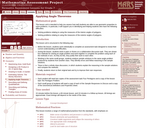Only Sharing Permitted
CC BY-NC-ND
Rating
0.0 stars

This lesson unit is intended to help you assess how well students are able to use geometric properties to solve problems. In particular, it will support you in identifying and helping students who have the following difficulties: Solving problems relating to using the measures of the interior angles of polygons; and solving problems relating to using the measures of the exterior angles of polygons.

Subject:
Geometry
Mathematics
Material Type:
Assessment
Lesson Plan
Provider:
Shell Center for Mathematical Education
Provider Set:
Mathematics Assessment Project (MAP)
06/24/2019Conditional Remix & Share Permitted
CC BY-NC-SA
Rating
0.0 stars

This book is a "flexed" version of CK-12's Basic Geometry that aligns with College Access Geometry and contains embedded literacy supports. It covers the essentials of geometry for the high school student.

Subject:
Mathematics
Material Type:
Textbook
Provider:
CK-12 Foundation
Provider Set:
CK-12 FlexBook
Author:
Fauteux, Michael
Zapata, Rosamaria
05/18/2011Conditional Remix & Share Permitted
CC BY-NC-SA
Rating
0.0 stars

CK-12 Foundation's Geometry FlexBook is a clear presentation of the essentials of geometry for the high school student. Topics include: Proof, Congruent Triangles, Quadrilaterals, Similarity, Perimeter & Area, Volume, and Transformations.

Subject:
Mathematics
Material Type:
Textbook
Provider:
CK-12 Foundation
Provider Set:
CK-12 FlexBook
10/06/2009Conditional Remix & Share Permitted
CC BY-NC-SA
Rating
0.0 stars

A structured geometry program teacher edition of daily lesson plans and teacher supports to accompany the College Access Reader: Geometry student edition.

Subject:
Mathematics
Material Type:
Textbook
Provider:
CK-12 Foundation
Provider Set:
CK-12 FlexBook
Author:
Fauteux, Michael
Zapata, Rosamaria
05/18/2011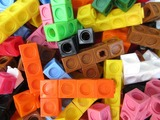Conditional Remix & Share Permitted
CC BY-NC-SA
Rating
0.0 stars

In this lesson students explore lines and angles in the context of a scavenger hunt.&nbsp;

Subject:
Mathematics
Material Type:
Activity/Lab
Lesson Plan
Author:
Drew Polly
07/25/2020Conditional Remix & Share Permitted
CC BY-NC-SA
Rating
0.0 stars

In this lesson students explore lines and angles in the context of North Carolina.&nbsp;

Subject:
Mathematics
Material Type:
Activity/Lab
Lesson Plan
Author:
Drew Polly
07/25/2020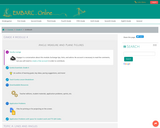Conditional Remix & Share Permitted
CC BY-NC-SA
Rating
0.0 stars

G4M4: Angle Measure and Plane Figures. Contains 16 Lessons.

Subject:
Mathematics
Material Type:
Module
Provider:
EMBARC.Online
08/13/2019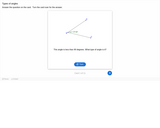Unrestricted Use
CC BY
Rating
0.0 stars

11 dialog cards with images, each card contains an angle type, description, and definition of different types of angles.

Subject:
Geometry
Mathematics
Material Type:
Interactive
Provider:
Michigan Virtual
07/19/2019Conditional Remix & Share Permitted
CC BY-NC-SA
Rating
0.0 stars

CK-12 Foundation's Geometry FlexBook is a clear presentation of the essentials of geometry for the high school student. Topics include: Proof, Congruent Triangles, Quadrilaterals, Similarity, Perimeter & Area, Volume, and Transformations.

Subject:
Mathematics
Material Type:
Textbook
Provider:
CK-12 Foundation
Provider Set:
CK-12 FlexBook
11/23/2010Conditional Remix & Share Permitted
CC BY-NC-SA
Rating
0.0 stars

CK-12's Geometry - Second Edition is a clear presentation of the essentials of geometry for the high school student. Topics include: Proofs, Triangles, Quadrilaterals, Similarity, Perimeter & Area, Volume, and Transformations.

Subject:
Mathematics
Material Type:
Textbook
Provider:
CK-12 Foundation
Provider Set:
CK-12 FlexBook
Author:
Dirga, Kathryn
Jordan, Lori
11/16/2010Conditional Remix & Share Permitted
CC BY-NC-SA
Rating
0.0 stars

CK-12 Geometry Teacher's Edition covers tips, common errors, enrichment, differentiated instruction and problem solving for teaching CK-12 Geometry Student Edition. The solution and assessment guides are available upon request.

Subject:
Mathematics
Material Type:
Textbook
Provider:
CK-12 Foundation
Provider Set:
CK-12 FlexBook
Author:
Kershaw, Jem
Kramer, Melissa
Zwack, Teresa
06/25/2011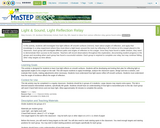Rating
0.0 stars

In this activity, students will investigate how light reflects off smooth surfaces (mirrors), learn about angles of reflection, and apply that knowledge in a relay experiment where they must direct a light beam around the room by reflecting it off 3 mirrors to hit a target placed in the classroom. Each group will investigate different paths and angles of reflection to hit a target. Once they have found a viable solution, they need to demonstrate their success to the teacher. Teachers will record observations throughout this exploration, documenting student progress in the groups and recording when each group has found a successful solution. Each group is required to complete one relay, but may continue hitting other relay targets as time allows.

Subject:
Science
Material Type:
Activity/Lab
Lesson Plan
Provider:
OER
Author:
Mary Kurvers, Minnesota Science Teachers Education Project
02/26/2019Conditional Remix & Share Permitted
CC BY-NC
Rating
0.0 stars

Four full-year digital course, built from the ground up and fully-aligned to the Common Core State Standards, for 7th grade Mathematics. Created using research-based approaches to teaching and learning, the Open Access Common Core Course for Mathematics&nbsp;is designed with student-centered learning in mind, including activities for students to develop valuable 21st century skills and academic mindset.

Subject:
Mathematics
Material Type:
Full Course
Provider:
Pearson
11/02/2020Conditional Remix & Share Permitted
CC BY-NC
Rating
0.0 stars

Constructions and Angles

Unit Overview

Type of Unit: Concept

Prior Knowledge

Students should be able to:

Use a protractor and ruler.
Identify different types of triangles and quadrilaterals and their characteristics.

Lesson Flow

After an initial exploratory lesson involving a paper folding activity that gets students thinking in general about angles and figures in a context, the unit is divided into two concept development sections. The first section focuses on types of angles—adjacent, supplementary, complementary, and vertical—and how they are manifested in quadrilaterals. The second section looks at triangles and their properties, including the angle sum, and how this affects other figures.

In the first set of conceptual lessons, students explore different types of angles and where the types of angles appear in quadrilaterals. Students fold paper and observe the angles formed, draw given angles, and explore interactive sketches that test many cases. Students use a protractor and ruler to draw parallelograms with given properties. They explore sketches of parallelograms with specific properties, such as perpendicular diagonals. After concluding the investigation of the angle types, students move on to the next set.

In the second set of conceptual development lessons, students focus on triangles. Students again fold paper to create figures and certain angles, such as complementary angles.

Students draw, using a protractor and ruler, other triangles with given properties. Students then explore triangles with certain known and unknown elements, such as the number of given sides and angles. This process starts with paper folding and drawing and continues with exploration of interactive sketches. Students draw conclusions about which cases allow 0, 1, 2, or an infinite number of triangles. In the course of the exploration, students discover that the sum of the measure of the interior angles of a triangle is 180°. They also learn that the sum of the measures of the interior angles of a quadrilateral is 360°. They explore other polygons to find their angle sum and determine if there is a relationship to angle sum of triangles. The exploration concludes with finding the measure of the interior angles of regular polygons and speculating about how this relates to a circle.

Lastly, students solve equations to find unknown angle measures. Using their previous experience, students find the remaining angle measures in a parallelogram when only one angle measure is given. Students also play a game similar to 20 Questions to identify types of triangles and quadrilaterals. Having completed the remaining lessons, students have a four-day Gallery to explore a variety of problems.

The unit ends with a unit assessment.

Subject:
Geometry
Mathematics
Provider:
Pearson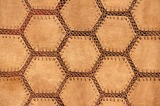Conditional Remix & Share Permitted
CC BY-NC
Rating
0.0 stars

Students learn more about the characteristics of parallelograms by folding paper and measuring the angles in a parallelogram. Students use a ruler and protractor to draw parallelograms with given properties. Then, students use a ruler and protractor to draw a rectangle.Key ConceptsOpposite angles of a parallelogram are congruent.Consecutive angles of a parallelogram are supplementary.Diagonals of a parallelogram bisect each other.Diagonals of a rectangle are congruent.Goals and Learning ObjectivesAccess prior knowledge of parallelograms.Understand that the sum of angle measures in any quadrilateral is 360°.Understand the relationship of the angles and diagonals in a parallelogram.Understand the relationship of the angles and diagonals in a rectangle.

Subject:
Geometry
Material Type:
Lesson Plan
Author:
Pearson
11/02/2020Conditional Remix & Share Permitted
CC BY-NC
Rating
0.0 stars

Students learn how the diagonals of a rhombus are related. They use interactive sketches to learn about the properties of the angles and diagonals of squares, rectangles, rhombuses, parallelograms, and other quadrilaterals.Key ConceptsThe sum of the measures of the angles of all quadrilaterals is 360°.The alternate angles (nonadjacent angles) of rhombuses and parallelograms have the same measure.The measure of the angles of rectangles and squares is 90°.The consecutive angles of parallelograms and rhombuses are supplementary. This applies to squares and rectangles as well.The diagonals of a parallelogram bisect each other.The diagonals of a rectangle are congruent and bisect each other.The diagonals of a rhombus bisect each other and are perpendicular.Goals and Learning ObjectivesMeasure the angles formed by the intersection of the diagonals of a rhombus.Explore the relationships of the angles of different squares, rectangles, rhombuses, parallelograms, and other quadrilaterals.Explore the relationships of the diagonals of different squares, rectangles, rhombuses, parallelograms, and other quadrilaterals.

Subject:
Geometry
Material Type:
Lesson Plan
Author:
Pearson